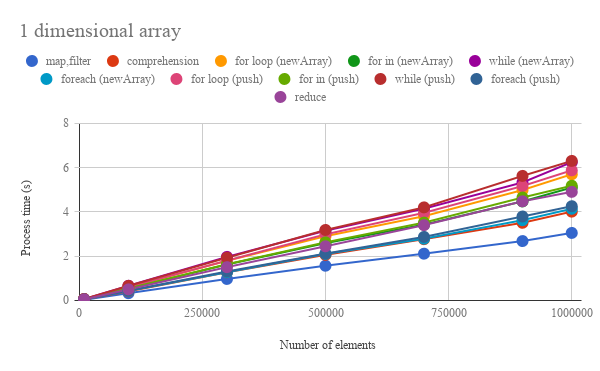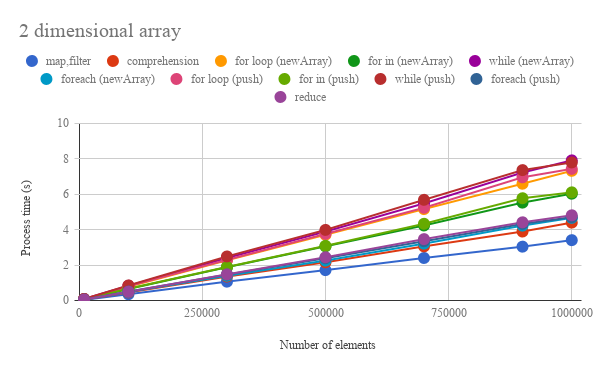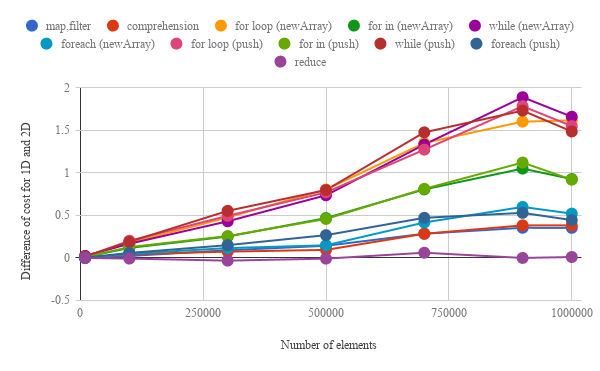Gists

# Benchmark: Loop for Array Processing using Google Apps Script

July 26, 2018 Updated. Result of reduce was added.

Kanshi Tanaike

# Introduction

Please be careful! This result can be only used for Google Apps Script.

There are a limit executing time for Google Apps Script (GAS). That is 6 minutes.1 So users always have to pay attention to reducing the process cost of the scripts. Especially, it is very important to know the process cost for the array processing, because the array processing is often used for spreadsheet and Google APIs. I have already reported “Improved Algorithms for Summation of Array Elements” as a method for reducing the process cost.2 In this report, the process cost of “loop” for the array processing using GAS has been investigated.

When users use the loop process for the array using GAS, the following 7 methods can be considered.

1. Normal “for loop”
• for (var i = 0; i < 10; i++) {array[i]}
2. for in
• for (var i in array) {array[i]}
3. while
• while (i < 10) {array[i++]}
4. forEach
• array.forEach(function(e){e})
5. map, filter
• array.map(function(e){e})
• array.filter(function(e){e})
6. Comprehension: GAS can use JavaScript 1.7.3 This means to be able to be used comprehension4 for GAS yet.
• [e for each (e in array)]
7. reduce
• array.reduce(function(ar,e){e},[])

As the result, it was found that the process costs of each method showed the large differences. For the array processing of both 1 dimensional and 2 dimensional array, “map, filter” and “while” were the lowest and the highest process cost, respectively. For processing 1 dimensional array and 2 dimensional array, “map, filter” could reduce the process cost for “while” by 50 % and 58 %, respectively. The ascending order of cost for each method was “map, filter”, “Comprehension”, “forEach”, “reduce”, “for in”, “for loop” and “while”. Also it was found that at GAS, the cost of push() and new Array() was almost the same. When the array was changed from 1 dimensional array to 2 dimensional array, the increasing ratio of the process cost for “Comprehension”, “forEach” and “map, filter” was much lower than that for “for in”, “for loop” and “while”. From these results, at GAS, it was found that “map, filter” is suitable for the array processing. For “reduce”, it was also found that the process costs between 1 and 2 dimensional array were almost the same.

# Experimental procedure

As the samples of array, 1 dimensional and 2 dimensional array were used. The array processing was performed by “for loop”, “for in”, “while”, “forEach”, “map, filter” and “Comprehension”. The process time was measured and the results were compared. Then, when we see the scripts for the array processing at various sites, push() is often used. As another way, there is the way for using new Array(). In this report, the process costs of push() and new Array() were also compared. In order to measure the process cost of array processing, the script which retrieves the multiple of 5 from the elements of arrays was used as a sample script. The sample scripts of Google Apps Scripts which were used for this report are here. a For measuring the process cost, the cost for creating array to use at the methods is not included. By the way, at GAS, the processing time is not stable as you know. So the average value for more than 100 times measurements was used for each data point which is shown by figures. At this time, the fluctuation of the average values was less than 1 %. I worry that each detailed-data point at my environment might be different from that at other user’s environment. But I think that the trend of this result can be used.

# ResultsFig 1. Number of elements vs. processing time for 1 dimensional array.Fig 2. Number of elements vs. processing time for 2 dimensional array.

Figures 1 and 2 show that the number of elements vs. processing time for 1 and 2 dimensional arrays, respectively. The results of push() and new Array() are also included in these figures. From both figures, it is found that the trend of all methods shows the linearly increase of process time for the number of elements under less than 1,000,000 elements. For the array processing of both 1 dimensional and 2 dimensional array, “map, filter” and “while” are the lowest and the highest process cost, respectively. For processing 1 dimensional array and 2 dimensional array, “map, filter” can reduce the process cost for “while” by 50 % and 58 %, respectively. The ascending order of cost for each method is “map, filter”, “Comprehension”, “forEach”, “reduce”, “for in”, “for loop” and “while”. Also it is found that at GAS, the costs of push() and new Array() were almost the same. This is the interesting result.Fig 3. Difference of process cost for 1D array and 2D array. This can be obtained by calculating the difference of each process time for between 1 dimensional array and 2 dimensional array. When the difference is closed to zero, it means that the process cost is difficult to be not changed, even when the dimension of array increases.

Table 1. Increasing ratio of process cost for 1D array and 2D array. This can be calculated from Fig. 3. For example, 7.3 % of comprehension means that when the array dimension is changed from 1D to 2D, the process cost will increase by 7.3 %.

Methods Increasing ratio (%)
reduce 0.3
comprehension 7.3
foreach (newArray) 9.8
map,filter 10.5
foreach (push) 12.7
for in (push) 19.7
for in (newArray) 20.4
for loop (push) 28.8
while (push) 28.9
while (newArray) 29.0
for loop (newArray) 30.7

When the array is changed from 1 dimensional array to 2 dimensional array, it is considered that the efficiency of the process cost is changed. The difference of the process cost was also investigated. Figure 3 shows the difference of the process cost for 1D array and 2D array. This can be obtained by calculating the difference of each process time for between 1 dimensional array and 2 dimensional array. When the difference is closed to zero, it means that the process cost is difficult to be not changed, even when the dimension of array increases. From Fig. 3, the increasing ratio for each method was calculated. Table 1 shows the increasing ratio. From Tab. 1, it is found that the the increasing ratio for “reduce”, Comprehension", “forEach” and “map, filter” is lower than that for “for in”, “for loop” and “while”. Especially, the increasing ratio of “reduce” is very low. This means that the process costs between 1 and 2 dimensional array are almost the same. This is one of the interesting results. From Figs. 1, 2 and Tab. 1, these results indicate that at GAS, the process cost for “reduce”, forEach", “Comprehension” and “map, filter” is lower than that for “for in”, “for loop” and “while”. And the results say that “map, filter” is suitable for the array processing.

I think that this result might be able to also propose that the conventional loop processing might be modified to new method. The sample scripts for the conventional method and the proposed method using this report are as follows.

var max = 1000;

// Conventional method
for (var i = 0; i < max; i++) {
// do something
}

// Proposed method
var r = Array.apply(null, new Array(max)).map(function(_, i) {
// do something
});


When the process costs for both methods were measured, it was found that proposed method could make the cost reduce about 10 % for the conventional method. Furthermore, the proposed method can also create an array, simultaneously. And from the results of this report, the cost of Array.apply() could be qualitatively estimate.

# Summary

In this report, the process cost of “loop” for the array processing using GAS has been investigated. As the result, it was found the following important features for GAS.

• In the case of the sample script for retrieving the multiple of 5 from the array, the loop using “map, filter” is the most suitable way.
• Ascending order of cost for each method is “map, filter”, “Comprehension”, “forEach”, “for in”, “for loop” and “while”.
• Cost for “forEach”, “Comprehension” and “map, filter” is lower than that for “for in”, “for loop” and “while”.
• Cost of push() and new Array() is almost the same.
• When the array is changed from 1 dimensional array to 2 dimensional array, the increasing ratio of the cost for “Comprehension”, “forEach” and “map, filter” is much lower than that for “for in”, “for loop” and “while”.
• For the conventional method using “for loop”, a new method could be proposed using the result of this report.
• For “reduce”, the process costs between 1 and 2 dimensional array are almost the same.

As a note, I have to describe that this is the result for Google Apps Script. For other languages, this result might be difference. And also, the process cost of this report might be modified by future update of Google.

# Appendix

## Scripts

### Creating sample arrays

The function for creating 1 dimensional array is as follows.

function make1dArray(row) {
var ar = [];
for (var i = 0; i < row; i++) {
ar[i] = i + 1;
}
return ar;
}


The function for creating 2 dimensional array is as follows.

function make2dArray(row) {
var ar1 = [];
for (var i = 0; i < row; i++) {
var ar2 = [];
for (var j = 0; j < 100; j++) {
ar2[j] = j + 1;
}
ar1[i] = ar2;
}
return ar1;
}


### For 1 dimensional array

// for loop using push()
var result = [];
for (var i = 0; i < array.length; i++) {
if (array[i] % 5 == 0) {
result.push(array[i]);
}
}

// for loop using new Array()
var result = new Array(array.length / 5);
var c = 0;
for (var i = 0; i < array.length; i++) {
if (array[i] % 5 == 0) {
result[c++] = array[i];
}
}

// for in using push()
var result = [];
for (var i in array) {
if (array[i] % 5 == 0) {
result.push(array[i]);
}
}

// for in using new Array()
var result = new Array(array.length / 5);
var c = 0;
for (var i in array) {
if (array[i] % 5 == 0) {
result[c++] = array[i];
}
}

// while using push()
var result = [];
var i = 0;
while (i < array.length) {
if (array[i] % 5 == 0) {
result.push(array[i]);
}
i += 1;
}

// while using new Array()
var result = new Array(array.length / 5);
var c = 0;
var i = 0;
while (i < array.length) {
if (array[i] % 5 == 0) {
result[c++] = array[i];
}
i += 1;
}

// forEach using push()
var result = [];
array.forEach(function(e) {
if (e % 5 == 0) {
result.push(e);
}
});

// forEach using new Array()
var result = new Array(array.length / 5);
var c = 0;
array.forEach(function(e) {
if (e % 5 == 0) {
result[c++] = e;
}
});

// map, filter
var result = array.filter(function(e) {return e % 5 == 0});

// comprehension
var result = [e for each (e in array) if (e % 5 == 0)];

// reduce
var result = array.reduce(function(o, e) {
if (e % 5 == 0) o.push(e);
return o;
}, []);


### For 2 dimensional array

// for loop using push()
var result = [];
for (var i = 0; i < array.length; i++) {
var temp = [];
for (var j = 0; j < array[i].length; j++) {
if (array[i][j] % 5 == 0) {
temp.push(array[i][j]);
}
}
result.push(temp);
}

// for loop using new Array()
var result = new Array(array.length);
for (var i = 0; i < array.length; i++) {
var temp = new Array(100 / 5)
var c = 0;
for (var j = 0; j < array[i].length; j++) {
if (array[i][j] % 5 == 0) {
temp[c++] = array[i][j];
}
}
result[i] = temp;
}

// for in using push()
var result = [];
for (var i in array) {
var temp = [];
for (var j in array[i]) {
if (array[i][j] % 5 == 0) {
temp.push(array[i][j]);
}
}
result.push(temp);
}

// for in using new Array()
var result = new Array(array.length);
var c1 = 0;
for (var i in array) {
var temp = new Array(100 / 5)
var c2 = 0;
for (var j in array[i]) {
if (array[i][j] % 5 == 0) {
temp[c2++] = array[i][j];
}
}
result[c1++] = temp;
}

// while using push()
var result = [];
var i = 0;
while (i < array.length) {
var temp = [];
var j = 0;
while (j < array[i].length) {
if (array[i][j] % 5 == 0) {
temp.push(array[i][j]);
}
j += 1;
}
result.push(temp);
i += 1;
}

// while using new Array()
var result = new Array(array.length);
var i = 0;
while (i < array.length) {
var temp = new Array(100 / 5)
var c = 0;
var j = 0;
while (j < array[i].length) {
if (array[i][j] % 5 == 0) {
temp[c++] = array[i][j];
}
j += 1;
}
result[i] = temp;
i += 1;
}

// forEach using push()
var result = [];
array.forEach(function(e) {
var temp = [];
e.forEach(function(f) {
if (f % 5 == 0) {
temp.push(f);
}
});
result.push(temp);
});

// forEach using new Array()
var result = new Array(array.length);
array.forEach(function(e, i) {
var temp = new Array(100 / 5)
var c = 0;
e.forEach(function(f) {
if (f % 5 == 0) {
temp[c++] = f;
}
});
result[i] = temp;
});

// map, filter
var result = array.map(function(e) {return e.filter(function(f) {return f % 5 == 0})});

// comprehension
var result = [[f for each (f in e) if (f % 5 == 0)] for each (e in array)];

// reduce
var result = array.reduce(function(o, e) {
var temp = e.reduce(function(p, f) {
if (f % 5 == 0) p.push(f);
return p;
}, []);
o.push(temp);
return o;
}, []);